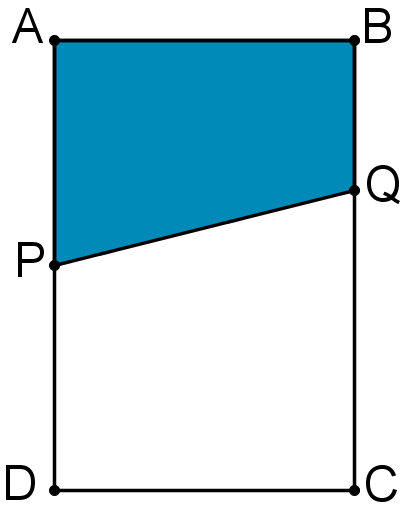#### You may also like### Framed

Seven small rectangular pictures have one inch wide frames. The frames are removed and the pictures are fitted together like a jigsaw to make a rectangle of length 12 inches. Find the dimensions of the pictures.### Tilted Squares

It's easy to work out the areas of most squares that we meet, but what if they were tilted?### Four or Five

The diagram shows a large rectangle composed of 9 smaller rectangles. If each of these rectangles has integer sides, what could the area of the large rectangle be?

# Guillotine

##### Age 11 to 14 ShortChallenge Level$ABCD$ is a rectangle. $P$ is the midpoint of $AD$; the length of $BQ$ is one third the length of $BC$. What fraction of the area of the rectangle is the area of the shaded quadrilateral $ABQP$?

If you liked this problem, here is an NRICH task which challenges you to use similar mathematical ideas.

This problem is taken from the UKMT Mathematical Challenges.
You can find more short problems, arranged by curriculum topic, in our short problems collection.